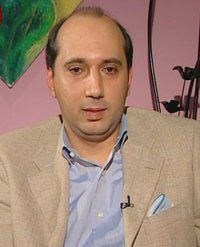# Hassan Ajami on the Unifulverse01/19/2023 - 20:27 PMProfessor Hassan Ajami

Hassan Ajami is a philosopher and scientific researcher who published twenty books in Arabic and five books in English, covering different topics, such as philosophy, literature, science, and culture. In his latest book “The Unifulverse: Our Transforming and Living Universe”, published by Lambert Academic publishing, the author Hassan Ajami developed a new hypothesis in cosmology and physics, according to which, uncertainty is equal to infinite probabilities divided by energy.

Ajami explains the content of this law in the following way: when the different probabilities are maximized, uncertainty is maximized, i.e., the greater number of different probabilities implies greater uncertainty, which indicates that the highest uncertainty amounts to infinite probabilities, while energy is needed to realize any probability.

This justifies the formulation of the previous law which defines uncertainty as being equal to the infinite probabilities divided by energy. Ajami relies on this law to explain how our actual universe could transform into any other universe from among all of the different and infinite parallel universes, leading to the unifulverse hypothesis, according to which, our actual universe is potentially full of all of the distinct parallel universes.

According to Ajami, since our actual universe is governed by uncertainty (such as the uncertainty principle of Heisenberg), and given that uncertainty is equal to infinite probabilities divided by energy, it follows that the uncertainty, governing our world, entails the infinite probabilities which are realized in different parallel universes, leading to the conclusion that all of the different parallel universes potentially exist in our actual universe.

Thus, it is possible for our actual universe to transform into any different universe from among the diverse parallel universes in light of the previous law. Ajami distinguishes between his scientific hypothesis and the classical theory of parallel universes because, according to this new cosmological hypothesis, the different parallel universes potentially exist in our actual universe, while, for the classical approach, the parallel universes exist in other dimensions parallel to our actual universe.

Ajami's new book “The Unifulverse” consists of thirteen chapters related to the unifulverse hypothesis. In these chapters, the author discusses diverse topics, such as reality and knowledge, and concludes that reality is a mathematical equation between the abstract and the concrete, while knowledge is a continuous process, instead of a static mental state. This book is useful for readers interested in science and philosophy, and widens the horizons of the interdisciplinary inquiries through its original scientific and philosophical ideas.